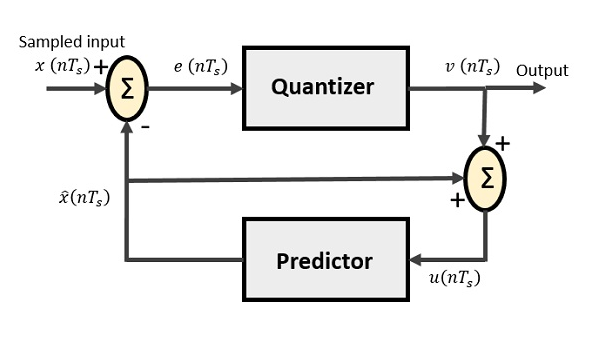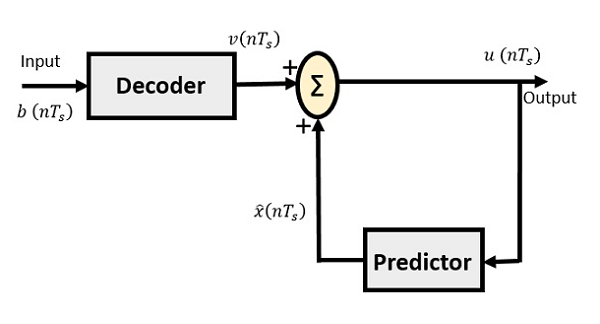# Digital Communication - Differential PCM

For the samples that are highly correlated, when encoded by PCM technique, leave redundant information behind. To process this redundant information and to have a better output, it is a wise decision to take a predicted sampled value, assumed from its previous output and summarize them with the quantized values. Such a process is called as Differential PCM (DPCM) technique.

## DPCM Transmitter

The DPCM Transmitter consists of Quantizer and Predictor with two summer circuits. Following is the block diagram of DPCM transmitter.The signals at each point are named as −

• $x(nT_{s})$ is the sampled input

• $\widehat{x}(nT_{s})$ is the predicted sample

• $e(nT_{s})$ is the difference of sampled input and predicted output, often called as prediction error

• $v(nT_{s})$ is the quantized output

• $u(nT_{s})$ is the predictor input which is actually the summer output of the predictor output and the quantizer output

The predictor produces the assumed samples from the previous outputs of the transmitter circuit. The input to this predictor is the quantized versions of the input signal $x(nT_{s})$.

Quantizer Output is represented as −

$$v(nT_{s}) = Q[e(nT_{s})]$$

$$= e(nT_{s}) + q(nT_{s})$$

Where q (nTs) is the quantization error

Predictor input is the sum of quantizer output and predictor output,

$$u(nT_{s}) = \widehat{x}(nT_{s}) + v(nT_{s})$$ $$u(nT_{s}) = \widehat{x}(nT_{s}) + e(nT_{s}) + q(nT_{s})$$ $$u(nT_{s}) = x(nT_{s}) + q(nT_{s})$$

The same predictor circuit is used in the decoder to reconstruct the original input.